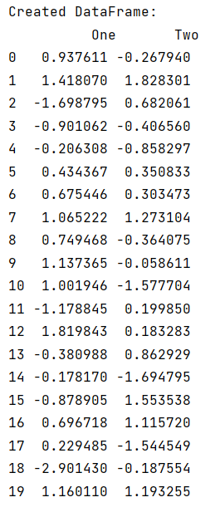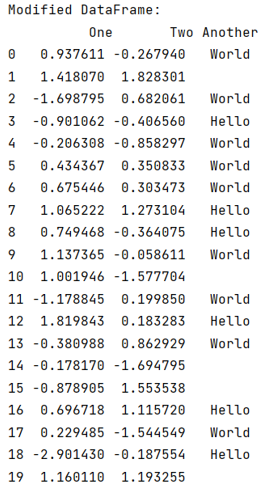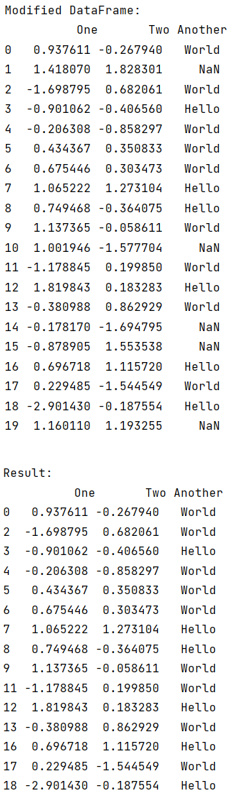# Drop rows containing empty cells from a pandas DataFrame

Given a Pandas DataFrame, we have to drop rows containing empty cells.
Submitted by Pranit Sharma, on July 01, 2022

Empty cells mean that at the time of creation or after importing a CSV file, some of the sells have an empty string.

Pandas is a special tool that allows us to analyze our data in such a way that it leads to a better understanding of data and helps us to create a strong machine learning model.

To drop rows that contain empty cells from a pandas DataFrame, we will first create a DataFrame that will be containing some empty strings, after that, we will replace these empty strings with NaN values followed by dropping the rows which contain NaN values by using dropna() method.

To work with pandas, we need to import pandas package first, below is the syntax:

```import pandas as pd
```

Let us understand with the help of an example,

## Python code to drop rows containing empty cells from a pandas DataFrame

```# Importing pandas package
import pandas as pd

# Importing numpy package
import numpy as np

# Creating a DataFrame
df = pd.DataFrame(np.random.randn(50, 2), columns=['One','Two'])

# Display DataFrame
print("Created DataFrame:\n",df,"\n")
```

Output:```# Adding another column
df['Another'] = np.random.choice(['Hello', '', 'World'], 20)

# Display modified DataFrame
print("Modified DataFrame:\n",df,"\n")
```

Output:```# Adding another column
df['Another'] = np.random.choice(['Hello', '', 'World'], 20)

# Display modified DataFrame
print("Modified DataFrame:\n",df,"\n")

# Replacing empty string with np.NaN
df['Another'] = df['Another'].replace('', np.nan)

# Display modified DataFrame:
print("Modified DataFrame:\n",df,"\n")

# Dropping rows where NaN is present
result = df.dropna(subset=['Another'])

# Display result
print("Result:\n",result)
```

Output:Languages: » C » C++ » C++ STL » Java » Data Structure » C#.Net » Android » Kotlin » SQL
Web Technologies: » PHP » Python » JavaScript » CSS » Ajax » Node.js » Web programming/HTML
Solved programs: » C » C++ » DS » Java » C#
Aptitude que. & ans.: » C » C++ » Java » DBMS
Interview que. & ans.: » C » Embedded C » Java » SEO » HR
CS Subjects: » CS Basics » O.S. » Networks » DBMS » Embedded Systems » Cloud Computing
» Machine learning » CS Organizations » Linux » DOS
More: » Articles » Puzzles » News/Updates